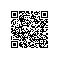# 浮点数类型在计算机里面的表示方法

Console.WriteLine("1.123f + 1.345f == 2.468f ? {0}",

1.123f + 1.345f == 2.468f); // False

Console.WriteLine("1.123f + 1.344f == 2.467f ? {0}",

1.123f + 1.344f == 2.467f); // True

Console.WriteLine("1.123 + 1.345 == 2.468 ? {0}",

1.123 + 1.345 == 2.468); // True

00000000 00000000 00110000 00111001

SEEEEEEE EMMMMMMM MMMMMMMM MMMMMMMM

M就是用来计算科学计数法里面的m，计算规则是，从左往右开始，第一个M代表2-1，第二个M表示2-2，依次类推。由于m要么就是大于1的小数，要么就是小于1的小数（记住，我们计数法里面的基数是，而不是10），如果e的值大于0，那么我们就可以加上这个1，如果e的值小于0，那么我们可以省略这个1，这是因为我们总是可以通过调整e的值来做到这一点。因此m里面小数点前面的1被省略掉了，这个省略掉的1可以根据e的值来推算出m的小数点前面到底有没有这个1

SEEEEEEE EEEEMMMM MMMMMMMM MMMMMMMM MMMMMMMM MMMMMMMM MMMMMMMM MMMMMMMM

double a = 1.345f;

double b = 1.123f;

double result = a + b;

double expected = 2.468f;

Console.WriteLine("result == expected ? {0}", result == expected);

11111111110101100001010001111011000000000000000000000000000000

00111111 11110101 10000101 00011110 11000000 00000000 00000000 00000000

(十进制的)1.0 + 0101 10000101 00011110 11000000 00000000 00000000 00000000所表示的小数

= 1.0 + 2**-2 + 2**-4 + 2**-5 + 2**-10 + 2**-12 + 2**-16 + 2**-17 + 2**-18 + 2**-19 + 2**-21 + 2**-22

= 1.0 + 0.25

+ 0.0625

+ 0.03125

+ 0.0009765625

+ 0.000244140625

+ 0.0000152587890625

+ 0.00000762939453125

+ 0.000003814697265625

+ 0.0000019073486328125

+ 0.000000476837158203125

+ 0.0000002384185791015625

= 1.0 + 0.3450000286102294921875

= 1.3450000286102294921875

1.344f + 1.123f == 2.467f，纯粹是巧合，因为前两者相加的值恰好等于后者在计算机里面的表现形式。使用钉钉扫一扫加入圈子
+ 订阅